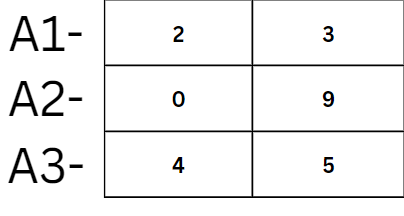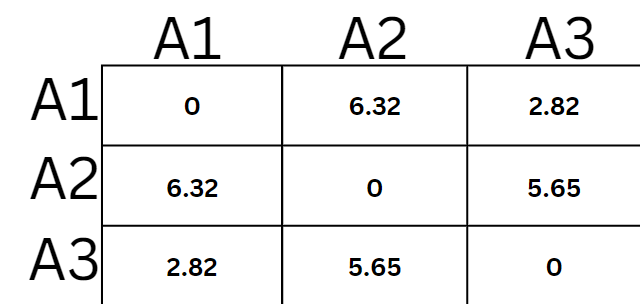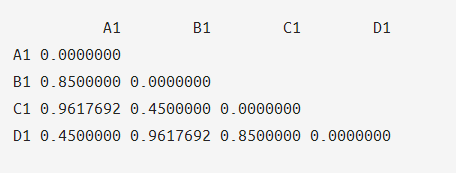Open in App
Not now

# Pairwise Distance Matrix in R

• Difficulty Level : Easy
• Last Updated : 30 Jan, 2023

A pairwise distance matrix is a 2-Dimensional matrix whose elements have the value of distances that are taken pairwise, hence the name Pairwise Matrix. This can be understood easily by the following example.

Let us consider a set of elements S1- {(2,3), (0,9), (4,5)}.If we calculate its Distance Matrix, we will get,The values of this Matrix have the pairwise euclidean distance between the items. The first row of this matrix contains the distance between A1 and with rest of the items i.e A2 and A3 including A1 too.

We can do pairwise distance calculations using R Programming Language too! We use the dist() function in the R programming language.  We can find manhattan or euclidean, pairwise distance.

## R

 `# Make three vectors``A1<-``c``(2,3)``A2<-``c``(0,9)``A3<-``c``(4,5)`` ` `#making a single matrix``CooR <- ``rbind``(A1, A2, A3)``dist``(CooR, method = ``"euclidean"``,``     ``diag = ``TRUE``, upper = ``TRUE``)`

Output:

```         A1       A2       A3
A1 0.000000 6.324555 2.828427
A2 6.324555 0.000000 5.656854
A3 2.828427 5.656854 0.000000```

This is our Pairwise Distance Matrix for the inputs {(2,3),(0,9),(4,5)}. Let’s try another example but this time using manhattan distance.

## R

 `# R program to calculate a pairwise distance matrix``# Make three vectors``A1<-``c``(1,1,7)``A2<-``c``(2,9,5)``A3<-``c``(9,6,3)`` ` `#making a single matrix``CooR <- ``rbind``(A1, A2, A3)``dist``(CooR, method = ``"manhattan"``,``     ``diag = ``TRUE``, upper = ``TRUE``)`

Output:

```   A1 A2 A3
A1  0 11 17
A2 11  0 12
A3 17 12  0    ```

Let us take another example for better understanding, we will calculate pairwise euclidean distance.

## R

 `# Making vectors to use for matrix`` ` `A1 <- ``c``(0, 0)``B1 <- ``c``(0.85, 0)``C1 <- ``c``(0.85, 0.45)``D1 <- ``c``(0, 0.45)`` ` `#making a single matrix``CooR <- ``rbind``(A1, B1, C1, D1)``dist``(CooR, method=``"euclidean"``, diag=``TRUE``, ``     ``upper=``FALSE``)`

Output:Pairwise Distance Matrix with just the lower triangular part

My Personal Notes arrow_drop_up